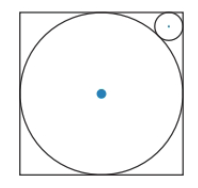Chapter 6.2, Problem 37EElementary Geometry For College St...

7th Edition
Alexander + 2 others
ISBN: 9781337614085

Solutions

Chapter
SectionElementary Geometry For College St...

7th Edition
Alexander + 2 others
ISBN: 9781337614085
Textbook Problem

The larger circle is inscribed in a square with sides of length 4 c m . The smaller circle is tangent to the larger circle and to two sides of the square, as shown. Find the length of the radius of the smaller circle.To determine

To find:

The length of the radius of the smaller circle.

Explanation

Given:

Calculation:

Consider the given figure.

The side lengths of the square are 4 centimetre.

This is the diameter of the inscribed larger circle.

Therefore, d=PQ=4cm.

Then r=XB=X0=XP=XQ=42=2cm.

Consider the square XOAP.

Here, side length is 2cm

Still sussing out bartleby?

Check out a sample textbook solution.

See a sample solution

The Solution to Your Study Problems

Bartleby provides explanations to thousands of textbook problems written by our experts, many with advanced degrees!

Get Started

In Exercises 2340, find the indicated limit. 29. limt1(2x33x2+x+2)

Applied Calculus for the Managerial, Life, and Social Sciences: A Brief Approach

In Problems 1-8, use a calculator to evaluate each expression. 8.

Mathematical Applications for the Management, Life, and Social Sciences

Simplify the expressions in Exercises 97106. (xy)1/3(yx)2/3

Finite Mathematics and Applied Calculus (MindTap Course List)

Evaluate the integral. 12(x2|x|)dx

Calculus (MindTap Course List)

True or False: xy + 6x2y = 10 x3 is linear.

Study Guide for Stewart's Single Variable Calculus: Early Transcendentals, 8th